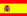You are here : > Home> Utilities > Mathematics & Statistics > Inverse trigonometric functions >

### Inverse Trigonometric Functions

This application calculates the inverse trigonometric functions : arc sine, arc cosine,   arc tangent,   arc cotangent, arc secant and arc cosecant, in degrees and radians.
Enter value as a real number, within the correspondent domains and ranges. Use a point (.) for decimal place. Example, 2.533 ; the results will be displayed automatically after you click on "Calculate".

 Inverse Trigonometric Functions Decimal places: Select:  arc sine arc cosine arc tangent arc cotangent arc secant arc cosecant Degrees: Radians: Note: